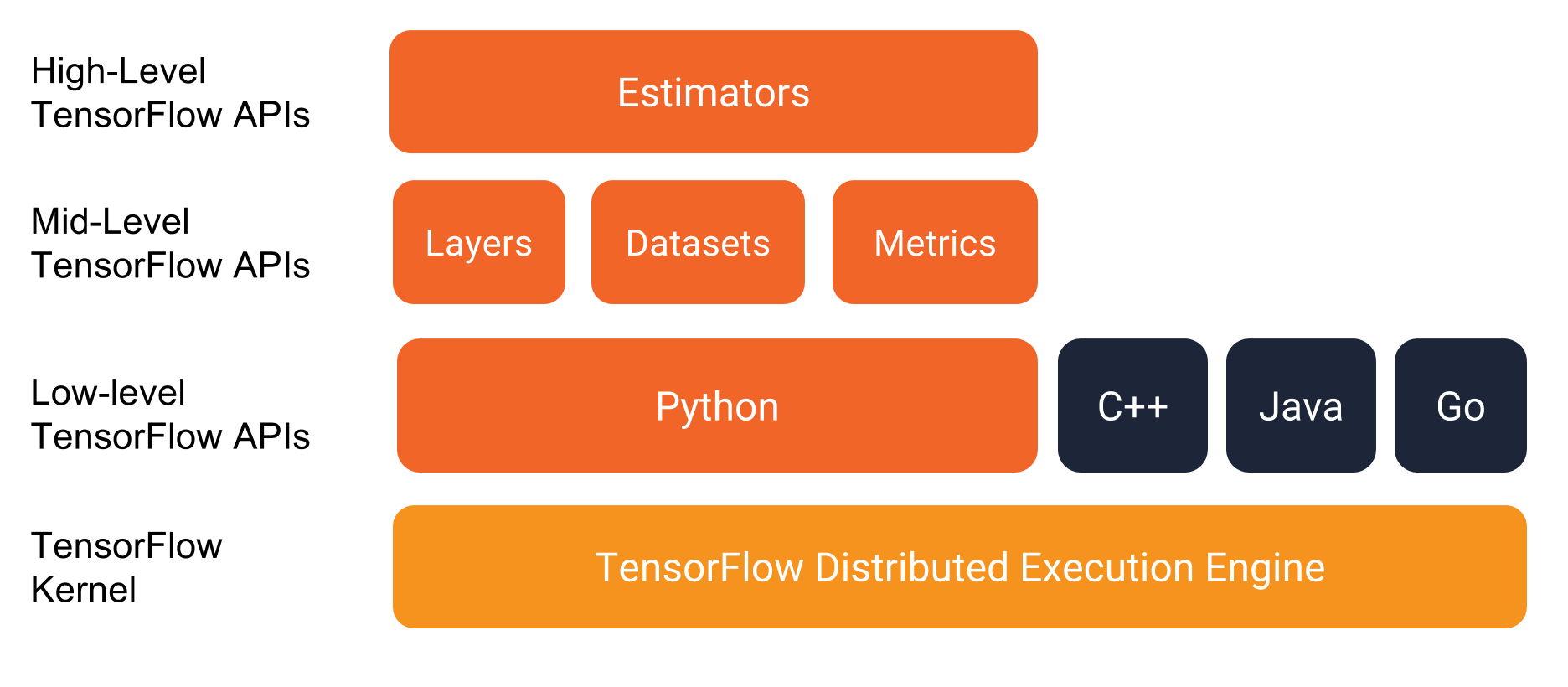# Graph Execution 入门

• 或多或少听说过机器学习
• 想学习编写 TensorFlow 程序
• 会使用 Python 编程

## 鸢尾花分类问题

• setosa 类
• virginica 类
• versicolor 类Iris 数据集的前 5 行如下：

6.4 2.8 5.6 2.2 2
5.0 2.3 3.3 1.0 1
4.9 2.5 4.5 1.7 2
4.9 3.1 1.5 0.1 0
5.7 3.8 1.7 0.3 0

• 最后一列 (种属) 被称为 标记（label）；前四列被称为 特征（feature）。特征用来形容样本数据，标记用于之后的结果预测。

• 一个 样本（example）包含所有特征的集合和样本的标记。上表中，5 条样本数据来自于一个数据量为 120 条数据的数据集。

• 0 对应 setosa
• 1 对应 versicolor
• 2 对应 virginica

## 运行示例程序前的准备工作

1. 安装 TensorFlow
2. 如果你是使用 virtualenv 或 Anaconda 安装 TensorFlow 的，初始化 TensorFlow 环境。
3. 安装/升级 pandas :

`pip install pandas`

1. 将 TensorFlow 模型 远程仓库从 github 克隆到本地，命令如下：

```````git clone https://github.com/tensorflow/models`
``````
2. 在该分支下，cd 到包含本文示例代码的目录下：

```````cd models/samples/core/get_started/`
``````

`get_started` 文件目录下，找到名为 `premade_estimator.py`的 python 文件。

## 运行示例程序

``````python premade_estimator.py
``````

``````...
Prediction is "Setosa" (99.6%), expected "Setosa"

Prediction is "Versicolor" (99.8%), expected "Versicolor"

Prediction is "Virginica" (97.9%), expected "Virginica"
``````

• 是否成功安装 TensorFlow ？
• 是否使用了正确版本的 TensorFlow ？程序`premade_estimators.py`需要版本号至少为 TensorFlow v1.4。
• 如果你通过 virtualenv 或 Anaconda 安装的 TensorFlow，是否初始化环境？

## TensorFlow 技术栈TensorFlow 编程环境

• Estimators
• Datasets

## 程序代码

• 引入数据集并解析
• 创建特征列描述数据
• 选择模型
• 训练模型
• 评估模型
• 使用训练后的模型进行预测。

### 引入数据集并解析

• 训练数据集`http://download.tensorflow.org/data/iris_training.csv`
• 测试数据集`http://download.tensorflow.org/data/iris_test.csv`

`premade_estimators.py` 程序通过 `load_data` 函数读取相邻路径的 `iris_data.py` 文件并解析为训练集和测试集。

``````# 定义数据 csv 文件地址

CSV_COLUMN_NAMES = ['SepalLength', 'SepalWidth',
'PetalLength', 'PetalWidth', 'Species']

...

"""Parses the csv file in TRAIN_URL and TEST_URL."""

# 新建路径本地训练集文件
train_path = tf.keras.utils.get_file(fname=TRAIN_URL.split('/')[-1],
origin=TRAIN_URL)
# 训练集路径为: ~/.keras/datasets/iris_training.csv

# 解析本地 CSV 文件
names=CSV_COLUMN_NAMES,  # 列
)
# 定义 train 变量为 DataFrame（pandas 库中类似表的数据结构）。

# 1. 定义变量 train_label 为样本标记，DataFrame 的最右行，
# 2. 从 DataFrame 中删除最右行，
# 3. 定义 DataFrame 中的剩余行为 train_features 样本特征。
train_features, train_label = train, train.pop(label_name)

# 对测试数据集执行上述操作
test_path = tf.keras.utils.get_file(TEST_URL.split('/')[-1], TEST_URL)
test_features, test_label = test, test.pop(label_name)

# 返回解析好的 DataFrame
return (train_features, train_label), (test_features, test_label)
``````

Keras 是一个开源机器学习库；`tf.keras` 是 TensorFlow 对 Keras 的实现。`premade_estimator.py` 程序只是 `tf.keras` 的一个函数入口，即： `tf.keras.utils.get_file` 方法，使拷贝远程 CSV 文件到本地系统更便捷。

``````    # 调用 load_data() 解析 CSV 文件
(train_feature, train_label), (test_feature, test_label) = load_data()
``````

Pandas 是一个开源的 Python 库，被用于 TensorFlow 函数中。Pandas 的DataFrame 是类似表的数据结构，每一列有列头，每一行有行标。下例为 `test_feature` DataFrame。

``````    SepalLength  SepalWidth  PetalLength  PetalWidth
0           5.9         3.0          4.2         1.5
1           6.9         3.1          5.4         2.1
2           5.1         3.3          1.7         0.5
...
27          6.7         3.1          4.7         1.5
28          6.7         3.3          5.7         2.5
29          6.4         2.9          4.3         1.3
``````

### 描述数据

`tf.feature_column.numeric_column`

``````# 为所有特征创建特征列
my_feature_columns = []
for key in train_x.keys():
my_feature_columns.append(tf.feature_column.numeric_column(key=key))
``````

``````my_feature_columns = [
tf.feature_column.numeric_column(key='SepalLength'),
tf.feature_column.numeric_column(key='SepalWidth'),
tf.feature_column.numeric_column(key='PetalLength'),
tf.feature_column.numeric_column(key='PetalWidth')
]
``````

### 选择模型类型

• 第一层有 4 个神经元，
• 第二次有 3 个神经元，
• 第三层有 2 个神经元。`tf.estimator.DNNClassifier`

``````    classifier = tf.estimator.DNNClassifier(
feature_columns=my_feature_columns,
hidden_units=[10, 10],
n_classes=3)
``````

``````        hidden_units=[10, 10],
``````

`hidden_units`列表的长度即隐藏层数（此处为 2 层）。列表中的每一个数值代表着该层神经元的个数（此处第一层有 10 个神经元，第二层有 10 个神经元）。只需简单地改变`hidden_units`的列表参数，就可以调试隐藏层数或神经元的个数。

`tf.Estimator.DNNClassifier` 的构造函数有一个可选参数 `optimizer` 优化器，在这里我们的程序没有声明。优化器 控制着模型怎样训练。当你在机器学习领域深入，优化器和学习率 （learning rate）将会变的很重要。

### 训练模型

``````    classifier.train(
input_fn=lambda:train_input_fn(train_feature, train_label, args.batch_size),
steps=args.train_steps)
``````

`steps`参数值指：通过多少次迭代后停止模型训练。`steps` 参数越大，意味着训练模型的时间越长。但训练模型时间越长，并不意味着模型更好。`args.train_steps` 的缺省值为 1000，训练的步骤数是一个可以调优的超参数。选择恰当的步骤数往往需要大量经验实践的积累。

`input_fn` 参数赋值为获得训练数据的函数，train 方法的调用通过 `train_input_fn` 函数获得训练数据。下面是该函数签名：

``````def train_input_fn(features, labels, batch_size):
``````

`train_input_fn` 传入下列参数值：

• `train_feature` 是一个 Python 的字典，该字典中：
• key 为样本特征名，
• value 为一个包含训练集所有样本值的数组
• `train_label` 为一个包含训练集所有样本标记的数组
• `args.batch_size` 数据类型为整型，定义了批量大小

`train_input_fn` 函数依赖于 Dataset API。这是一个高层 TensorFlow API，用于读取数据并转化成 `train` 方法所需的格式。

``````    dataset = tf.data.Dataset.from_tensor_slices((dict(features), labels))
``````

`tf.dataset` 类给训练提供了许多有用的预备样本。比如下面 3 个函数:

``````    dataset = dataset.shuffle(buffer_size=1000).repeat(count=None).batch(batch_size)
``````

`train` 方法每次批量处理样本，都通过`tf.data.Dataset.batch`方法串联多个样本创建一个批处理。我们程序中设置默认 批量大小 为 100，意味着 `batch` 方法串联几组数量为 100 的样本。理想的批量大小取决于问题本身，根据经验法则，小批量往往可以使 `train` 方法更快地训练模型，但有时候要付出准确率下降的代价。

`return` 返回一批样本给调用方法（`train` 方法）。

``````   return dataset.make_one_shot_iterator().get_next()
``````

### 评估模型

5.9 3.0 4.3 1.5 1 1
6.9 3.1 5.4 2.1 2 2
5.1 3.3 1.7 0.5 0 0
6.0 3.4 4.5 1.6 1 2
5.5 2.5 4.0 1.3 1 1

``````# 评估模型
eval_result = classifier.evaluate(
input_fn=lambda:eval_input_fn(test_x, test_y, args.batch_size))

print('\nTest set accuracy: {accuracy:0.3f}\n'.format(**eval_result))
``````

``````def eval_input_fn(features, labels=None, batch_size=None):
"""An input function for evaluation or prediction"""
if labels is None:
# 无标记，仅使用特征
inputs = features
else:
inputs = (features, labels)

# 转换输入为 tf.dataset 对象
dataset = tf.data.Dataset.from_tensor_slices(inputs)

# 批量处理样本
assert batch_size is not None, "batch_size must not be None"
dataset = dataset.batch(batch_size)

# 返回流程的读结尾
return dataset.make_one_shot_iterator().get_next()
``````

1. 处理测试集数据，将特征和标记转化为 `tf.dataset` 对象。
2. 创建一批测试集样本（测试集样本不需要洗牌或重复随机化）。
3. 返回测试集样本给 `classifier.evaluate`

``````Test set accuracy: 0.967
``````

### 预测

``````    predict_x = {
'SepalLength': [5.1, 5.9, 6.9],
'SepalWidth': [3.3, 3.0, 3.1],
'PetalLength': [1.7, 4.2, 5.4],
'PetalWidth': [0.5, 1.5, 2.1],
}
``````

``````predictions = classifier.predict(
input_fn=lambda:eval_input_fn(predict_x,
labels=None,
batch_size=args.batch_size))
``````

`evaluate` 方法一样，`predict` 方法通过 `eval_input_fn` 收集样本。

1. 将我们刚刚人造的 3-元素 数据集特征转换。
2. 从刚才的数据集中创建批量的 3 个样本。
3. 返回批量的样本给 `classifier.predict`

`predict` 方法返回了一个 python iterable 对象，以字典结构输出每个样本的预测结果。该字典包含多个键值对。`probabilities` 的值是一个包含 3 个浮点值的列表，每个浮点值代表输入样本是该鸢尾花种属的可能性。例如，下面这个 `probabilities` 列表：

``````'probabilities': array([  1.19127117e-08,   3.97069454e-02,   9.60292995e-01])
``````

• 该鸢尾花样本是 Setosa 的概率忽略不计。
• 有 3.97% 概率为 Versicolor 类。
• 有 96.0% 概率为 Virginica 类。

`class_ids` 的值为仅有一个元素的数组，表明该样本最有可能是哪个种类：

``````'class_ids': array()
``````

`2` 类对应 Virginica 类鸢尾花。下面代码迭代整个 `predictions` 并针对每个 `predictions` 生成报告：

``````for pred_dict, expec in zip(predictions, expected):
template = ('\nPrediction is "{}" ({:.1f}%), expected "{}"')

class_id = pred_dict['class_ids']
probability = pred_dict['probabilities'][class_id]
print(template.format(iris_data.SPECIES[class_id], 100 * probability, expec))
``````

``````...
Prediction is "Setosa" (99.6%), expected "Setosa"

Prediction is "Versicolor" (99.8%), expected "Versicolor"

Prediction is "Virginica" (97.9%), expected "Virginica"
``````